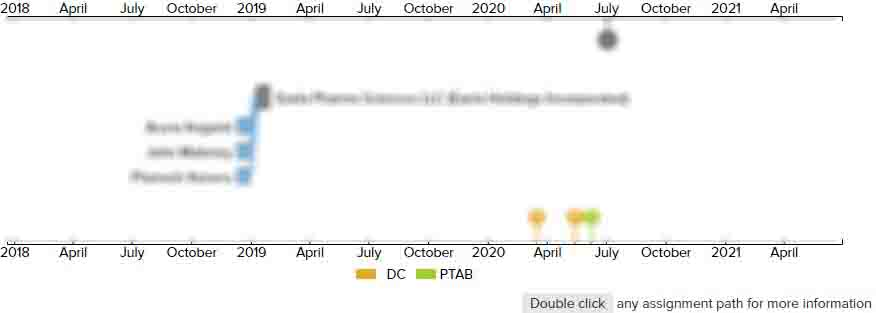# Method and system for matching speech data

• US 7,707,032 B2
• Filed: 10/20/2005
• Issued: 04/27/2010
• Est. Priority Date: 10/20/2005
• Status: Active Grant
##### First Claim
Patent Images

1. A method for matching speech data used to determine the similarity between an input speech data and a sample speech data, the method comprising:

• segmenting the input speech data into a plurality of input speech frames;

segmenting the sample speech data into a plurality of sample speech frames;

building a matching matrix, wherein each element of the matching matrix corresponds to one of the input speech frames and one of the sample speech frames and indicates a distance value between the corresponding input speech frame and the corresponding sample speech frame;

determining a minimum value of the distance values indicated in each row of elements of the matching matrix, thereby obtaining a plurality of minimum distance values of the respective rows of elements of the matching matrix, determining a second least value of the distance values indicated in each row of elements of the matching matrix, thereby obtaining a plurality of second least distance values of the respective rows of elements of the matching matrix;

summing up the minimum distance values and the second least distance value of the distance values indicated in each row of elements of the matching matrix, thereby obtaining a row score, determined by;

determining a minimum value of the distance values indicated in each column of elements of the matching matrix, thereby obtaining a plurality of another minimum distance values of the respective columns of elements of the matching matrix, determining a second least value of the distance values indicated in each column of elements of the matching matrix, thereby obtaining a plurality of second least distance values of the respective columns of elements of the matching matrix;

summing up the another minimum distance values and the second least value of the indicated distance values in each column of elements of the matching matrix distance values, thereby obtaining a column score, wherein;

calculating a matching score obtained by combining the distance row score and the column score; and

determining whether the input speech data and the sample speech data are similar according to the matching score.

• ##### 1 Assignment
Timeline View
Assignment View×
×